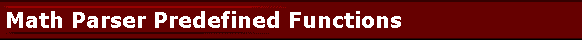## Predefined Functions of bcParser.NET Math Parser Component

Following functions are predefined in the bcParser.NET Math Expression Parser Component. You can remove them or you can add your own as needed at runtime.

SQR: Square function which can be used as SQR(X)

SIN: Sinus function which can be used as SIN(X), X is a real-type expression. Sin returns the sine of the angle X in radians.

COS: Cosinus function which can be used as COS(X), X is a real-type expression. COS returns the cosine of the angle X in radians.

ATAN: ArcTangent function which can be used as ATAN(X)

SINH: Sinus Hyperbolic function which can be used as SINH(X)

COSH: Cosinus Hyperbolic function which can be used as COSH(X)

COTAN: which can be used as COTAN(X)

TAN: which can be used as TAN(X)

EXP: which can be used as EXP(X)

LN: natural log, which can be used as LN(X)

LOG: 10 based log, which can be used as LOG(X)

SQRT: which can be used as SQRT(X)

ABS: absolute value, which can be used as ABS(X)

SIGN: SIGN(X) returns -1 if X<0; +1 if X>0, 0 if X=0; it can be used as SQR(X)

TRUNC: Discards the fractional part of a number. e.g. TRUNC(-3.2) is -3, TRUNC(3.2) is 3.

CEIL: CEIL(-3.2) = 3, CEIL(3.2) = 4

FLOOR: FLOOR(-3.2) = -4, FLOOR(3.2) = 3

VAL: VAL("3.1") = 3.1 Returns the floating point numeric value of the string argument.

Predefined functions that take two parameters are:

INTPOW: The INTPOW function raises Base to an integral power. INTPOW(2, 3) = 8. Note that result of INTPOW(2, 3.4) = 8 as well.

POW: The Power function raises Base to any power. For fractional exponents or exponents greater than MaxInt, Base must be greater than 0.

LOGN: The LogN function returns the log base N of X. Example: LOGN(10, 100) = 2

MIN: MIN(2, 3) is 2.

MAX: MAX(2, 3) is 3.

IF: The IF(b, case1, case2) function provides branching capability. If b is not 0, then it returns case1, else it returns case2. Behavior is similar to C#'s: return b ? case1 : case2;
If b==0 then case1 will not be Evaluated, and vice versa. Example: IF(HEIGHT, 3/HEIGHT, 3) will make sure 3/HEIGHT does not cause division by zero.

Predefined functions that take no parameters are: RND: RND() function generates a random number (double value) between 0 and 1.

STR: STR(123) function returns the string representation of the passed value: "123".

SUBSTR: SUBSTR("Hello", 1,3) function returns the substring just like C# String.Substring function. The first parameter is the string, the second parameter is which index (0 based) to start copying, and the last parameter is the number of characters to copy. For example, SUBSTR("Hello", 1,3) returns "ell".

STRLEN: STRLEN("abc") function returns the length of the string parameter. For example, for "abc" it returns 3.

CONCAT: CONCAT("abc","def",...) function returns the concatanated strings: "abcdef". There is no preset limit on the number of parameters.

TRIM: TRIM(" abc ") function returns the trimmed version of the string parameter: " abc " -> "abc".

SUM: SUM(2,3,5,...) functions returns the sum of it's arguments. There is no preset limit on the number of parameters.

User functions can be added using CreateFunc method. Functions and Variables can be deleted using DeleteVar, DeleteFunc, DeleteAllVars, DeleteAllFuncs methods.

webmaster@gobestcode.com